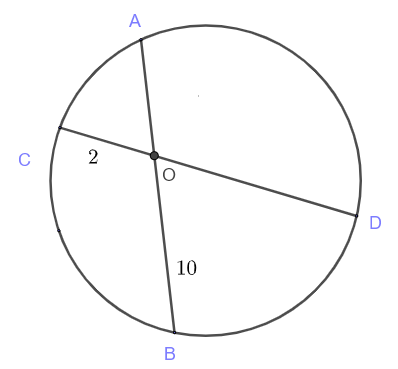# Intersecting Chords Theorem Questions with Solutions

Consider the circle with chords $A B$ and $E D$.The intersecting chords theorem  states that for any two chords $A B$ and $E D$ that intersects at the point $O$, we have $OA \times OB = OE \times OD$

## Examples With Solutions

Question 1
Find $x$ in the diagram below.Solution
Apply the intersecting chords theorem to $AB$ and $ED$ to write: $\quad OA \times OB = OE \times OD$
Substitute the known quantities: $\quad 2 \times 5 = 6 \times x$
Solve for $x$: $\quad x = \dfrac{10}{6} = \dfrac{5}{3}$

Question 2
Find $x$ and $y$ in the diagram below.Solution
Apply the intersecting chords theorem to $AB$ and $CD$ to write: $\quad OA \times OB = OD \times OC$
Substitute the known quantities: $\quad 7 \times 10 = 12 \times x$
Solve for $x$: $\quad x = \dfrac{70}{12} = \dfrac{35}{6}$

Apply the intersecting chords theorem to $AB$ and $EF$ to write: $\quad OA \times OB = OF \times OE$
Substitute the known quantities: $\quad 7 \times 10 = 11 \times y$
Solve for $y$: $\quad y = \dfrac{70}{11}$

Question 3
Find $x$ in the diagram below.Solution
Apply the intersecting chords theorem to $AB$ and $CD$ to write: $\quad OA \times OB = OD \times OC$
Substitute the known quantities: $\quad 10 \times (2x-1) = (2x+3) \times 3$
Expand: $\quad 20x - 10 = 6x + 9$
Rewrite the above equation with terms in $x$ on one side of the equation: $\quad 20x - 6x = 9 + 10$
Group and solve for $x$: $\quad x = \dfrac{19}{14}$

Question 4
Find $x$ in the diagram below.Solution
Apply the intersecting chords theorem to $AB$ and $CD$ to write: $\quad OA \times OB = OD \times OC$
Substitute by the expressions in $x$ : $\quad (x-1) \times (x+6) = (2x+3) \times (x-2)$
Expand: $\quad x^2+5x-6 = 2x^2-x-6$
Rewrite the above equation with standard form: $\quad x^2-6x = 0$
Factor the right side: $\quad x(x-6) = 0$
Solve for $x$ to obtain two solutions: $\quad x = 0$ and $x = 6$.
If you substitute $x = 0$ in the given algebraic expressions $OC = x - 2$, that will give $OC = - 2$ a negative length which is not allowed.
Hence the only valid solution is $x = 6$.

Question 5
What is the ratio $r$ of the area of triangle $OBD$ to the area of triangle $OCA$?Solution
We use the sine rule formula for the area of a triangle.
Area of $\; \triangle OBD = \dfrac{1}{2} \times OD \times 10 \times \sin \angle BOD$
Area of $\; \triangle OCA = \dfrac{1}{2} \times 2 \times OA \times \sin \angle COA$
The ratio $r$ is given by: $\quad r = \dfrac{\dfrac{1}{2} \times OD \times 10 \times \sin \angle BOD}{\dfrac{1}{2} \times 2 \times OA \times \sin \angle COA }$
Angles $\angle BOD$ and $\angle COA$ have equal sizes because they are vertical angles and therefore $\sin \angle BOD = \sin \angle COA$
We now simplify the expression of $r$: $\quad r = \dfrac{ OD \times 10 }{2 \times OA } = 5 \dfrac{OD}{OA}$
Apply the intersecting chords theorem to $AB$ and $CD$ to write: $\quad OA \times 10 = OD \times 2$
Hence : $\quad \dfrac{OD}{OA} = \dfrac{10}{2} = 5$
Substitute to obtain: $\quad r = 5 \times 5 = 25$